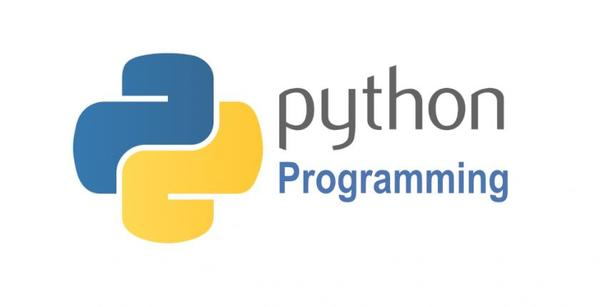## Oldest trick in the programmingBefore half-century, each bit inside of the computer was important. One of the most frequently used function was "swap".

Swap do the simple thing and you can say well I know. But in that time each operation and memory usage was important. So to have an efficient swap between 2 variables and not using third variables.

So trick goes like this:

```A = A + B
B = A - B
A = A - B
```

```TMP = A
A = B
B = TMP
```

Python code (tnx to Jovan Sh):

```>>> a = 5
>>> b = 3
>>> (a, b) = (b, a)
>>> print a,b
3 5
```

With this approach at that time, programmers save a lot of memory usage.

Also, you can find all these tricks in the book Hacker's Delight